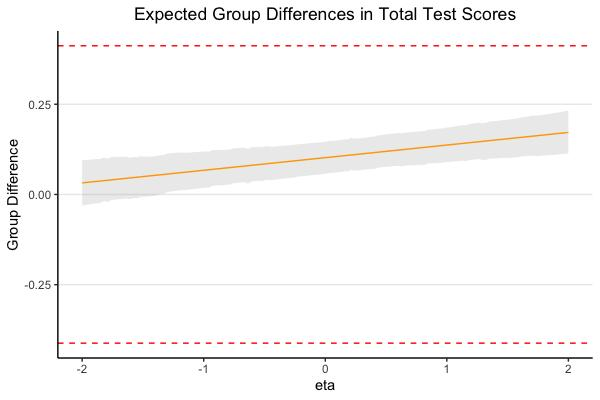# A Bayesian region of measurement equivalence (ROME) approach for establishing measurement invariance### Abstract

Measurement invariance research has focused on identifying biases in test indicators measuring a latent trait across two or more groups. However, relatively little attention has been devoted to the practical implications of noninvariance. An important question is whether noninvariance in indicators or items results in differences in observed composite scores across groups. The current study introduces the Bayesian region of measurement equivalence (ROME) as a framework for visualizing and testing the combined impact of partial invariance on the group difference in observed scores. Under the proposed framework, researchers first compute the highest posterior density intervals (HPDIs)—which contain the most plausible values—for the expected group difference in observed test scores over a range of latent trait levels. By comparing the HPDIs with a predetermined range of values that is practically equivalent to zero (i.e., region of measurement equivalence), researchers can determine whether a test instrument is practically invariant. The proposed ROME method can be used for both continuous indicators and ordinal items. We illustrated ROME using five items measuring mathematics-specific self-efficacy from a nationally representative sample of 10th graders. Whereas conventional invariance testing identifies a partial strict invariance model across gender, the statistically significant noninvariant items were found to have a negligible impact on the comparison of the observed scores. This empirical example demonstrates the utility of the ROME method for assessing practical significance when statistically significant item noninvariance is found.

Type
Publication
Psychological Methods. Advance Online Publication